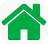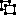Home

Personal
Webcam
Biography
Contact Me

GBIC >> FreeWare >> gbAnimator >> Math
 gbAnimator - MathTo exactly calculate the position and velocity of a body of masses connected by springs requires the simultaneous solution of multiple equations. However, by using a technique called finite element modeling, a close approximation of the solution can be achieved. That is the approach used by gbAnimator. For each node in the model the effects of all forces (gravity, wind, and gravitational nodes) are calculated. Added to that are the effects of any link attached to the node. These calculations are made over small time intervals. The basic equations used are: Force exerted by a spring Force = K * L = spring constant * (current length - rest length) The force is exerted by the spring on the mass at each end of the spring when the spring is either compressed or stretched beyond rest position). Acceleration due to spring force Acceleration = Force / Mass The forces applied to a mass attached to the end of the spring result in an acceleration of the mass. The acceleration is added to the acceleration of gravity to describe the motion of the masses. Position of a mass as a function of the applied acceleration Position = xo + vo*t + .5*a*t^2 where xo is starting position and vo is starting velocity In the case of gbAnimator, the acceleration is due to gravity and to the springs (natural force plus animator force). Another force gbAnimator takes into account is friction, which results in a loss of tangential velocity when a mass comes in contact with a surface. Force exerted by wind Force = w Each node feels a force from the wind. This force can be increased, corresponding the an increase in wind. In real life, the force of wind depends on the shape and cross-section of the object being hit by the wind. gbAnimator assumes that all node are identical and experience the same wind force. The force on each node Force exerted by the environment Force = -c * velocity The environment of gbAnimator can be thought of a fluid, such as air or liquid, where the resistance of movement through the fluid is determined by the viscosity setting. At zero viscosity, no resistance is felt. As viscosity is raised, resistance is increased and movemement becomes more difficult. This has the effect of slowing gbAnimation speed in all directions. Force exerted by gravitational nodes Force = g / d^2 where g is the gravitational force of the object and d is the distance between the two nodes. In this case, gbAnimator does not exactly follow real-world physics, where calculation of the movement due to gravity must take into account the mass of the two objects. A simpler model was chosen, where a user can select a force (g) that applies at a distance of 1, and then that force diminishes as the square of the distance to another node. This gives the effect that a node can attract other nodes, more strongly as the distance between them is reduced.